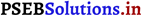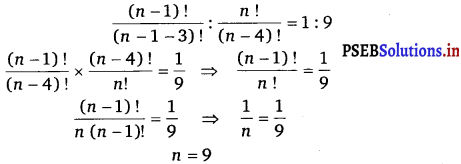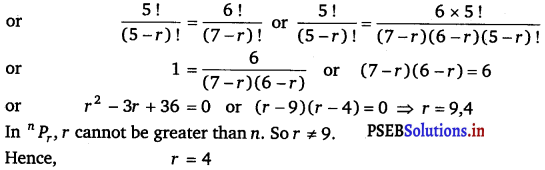# PSEB 11th Class Maths Solutions Chapter 7 Permutations and Combinations Ex 7.3

Punjab State Board PSEB 11th Class Maths Book Solutions Chapter 7 Permutations and Combinations Ex 7.3 Textbook Exercise Questions and Answers.

## PSEB Solutions for Class 11 Maths Chapter 7 Permutations and Combinations Ex 7.3

Question 1.
How many 3-digit numbers can be formed by using the digits 1 to 9 if no digit is repeated?
3-digit numbers have to be formed using the digits 1 to 9.
Here, the order of the digits matters.
Therefore, there will be as many 3-digit numbers as there are permutations of 9 different digits taken 3 at a time.
Therefore, required number of 3-digit numbers
= $${ }^{9} \mathrm{P}_{3}=\frac{9 !}{(9-3) !}=\frac{9 !}{6 !}$$

= $$\frac{9 \times 8 \times 7 \times 6 !}{6 !}$$

= 9 × 8 × 7 = 504.

Question 2.
How many 4-digit numbers are there with no digit repeated?
The thousands place of the 4-digit number is to be filled with any of the digits from 1 to 9 as the digit 0 cannot be included. Therefore, the number of ways in which thousands place can be filled is 9.

The hundreds, tens, and units place can be filled by any of the digits from 0 to 9. However, the digits cannot be repeated in the 4-digit numbers and thousands place is already occupied with a digit. The hundreds, tens, and units place is to be filled by the remaining 9 digits. Therefore, there will be as many such 3-digit numbers as there are permutations of 9 different digits taken 3 at a time.
Number of such 3-digit numbers
= $${ }^{9} \mathrm{P}_{3}=\frac{9 !}{(9-3) !}=\frac{9 !}{6 !}$$

= $$\frac{9 \times 8 \times 7 \times 6 !}{6 !}$$

= 9 × 8 × 7 = 504.
Thus, by multiplication principle, the required number of 4-digit numbers is 9 × 504 = 4536.Question 3.
How many 3-digit even numbers can be made using the digits 1, 2, 3, 4, 6, 7, if no digit is repeated?
3-digit even numbers are to be formed using the given six digits, 1,2,3,4, 6, and 7, without repeating the digits. Then, units digits can be filled in 3 ways by any of the digits, 2, 4, or 6.

Since the digits cannot be repeated in the 3-digit numbers and units place is already occupied with a digit (which is even), the hundreds and tens place is to be filled by the remaining 5 digits.

Therefore, the number of ways in which hundreds and tens place can be filled with the remaining 5 digits is the permutation of 5 different digits taken 2 at a time.

Number of ways of filling hundreds and tens place = $${ }^{5} P_{2}=\frac{5 !}{(5-2) !}$$

= $$\frac{5 !}{3 !}=\frac{5 \times 4 \times 3 !}{3 !}$$ = 20
Thus, by multiplication principle, the required number of 3-digit numbers is 3 × 20 = 60.

Question 4.
Find the number of 4-digit numbers that can be formed using the digits 1, 2, 3, 4, 5 if no digit is repeated. How many of these will be even?
4-digit numbers are to be formed using the digits 1, 2, 3, 4, and 5.
There will be as many 4-digit numbers as there are permutations of 5 different digits taken 4 at a time.
Therefore, required number of 4 digit numbers
= $$={ }^{5} P_{4}=\frac{5 !}{(5-4) !}=\frac{5 !}{1 !}$$
= 5 × 4 × 3 × 2 × 1 =120

Among the 4-digit numbers formed by using the digits 1, 2, 3, 4, 5, even numbers end with either 2 or 4.
The number of ways in which units place is filled with digits is 2.

Since the digits are not repeated and the units place is already occupied with a digit (which is even), the remaining places are to be filled by the remaining 4 digits.

Therefore, the number of ways in which the remaining places can be filled is the permutation of 4 different digits taken 3 at a time.
Number of ways of filling the remaining places = $${ }^{4} P_{3}=\frac{4 !}{(4-3) !}=\frac{4 !}{1 !}$$
= 4 × 3 × 2 × 1 = 24

Thus, by multiplication principle, the required number of even numbers is 24 × 2 =48.Question 5.
From a committee of 8 persons, in how many ways can we choose a chairman and a vice chairman assuming one person cannnot hold more than one position?
From a committee of 8 persons, a chairman and a vice chairman are to be chosen in such a way that one person cannot hold more than one position.
Here, the number of ways of choosing a chairman and a vice chairman is the permutation of 8 different objects taken 2 at a time.
Thus, required number of ways = $${ }^{8} P_{2}=\frac{8 !}{(8-2) !}=\frac{8 !}{6 !}$$

= $$\frac{8 \times 7 \times 6 !}{6 !}$$

= 8 × 7 = 56

Question 6.
Find n if $${ }^{n-1} \mathbf{P}_{3}:{ }^{n} \mathbf{P}_{\mathbf{4}}$$ = 1 : 9.
we have $${ }^{n-1} \mathbf{P}_{3}:{ }^{n} \mathbf{P}_{\mathbf{4}}$$ = 1 : 9Question 7.
Find r if
(i) $${ }^{5} \mathbf{P}_{\mathrm{r}}=\mathbf{2}^{\mathbf{6}} \mathbf{P}_{\mathrm{r}-1}$$

(ii) $${ }^{5} \mathbf{P}_{\mathbf{r}}={ }^{6} \mathbf{P}_{\mathrm{r}-1}$$
(i) $${ }^{5} \mathbf{P}_{\mathrm{r}}=\mathbf{2}^{\mathbf{6}} \mathbf{P}_{\mathrm{r}-1}$$

or $$\frac{5 !}{(5-r) !}=2 \times \frac{6 !}{[6-(r-1)] !}=\frac{2 \times 6 !}{(7-r) !}$$

or $$\frac{5 !}{(5-r) !}=\frac{2 \times 6 \times 5 !}{(7-r)(6-r)(5-r) !}$$

or 1 = $$\frac{12 !}{(7-r)(6-r)}$$

or (7 – r) (6 – r) = 12
or r2 – 13r + 30 = 0
(r – 10) (r – 3) = 0
r = 10, 3
If $${ }^{5} \mathbf{P}_{\mathrm{r}}$$, r cannot be greater than 5. So r ≠ 10.
Hence, r = 3.

(ii) $${ }^{5} P_{r}={ }^{6} P_{r-1}$$

⇒ $$\frac{5 !}{(5-r) !}=\frac{6 !}{[6-(r-1)] !}$$Question 8.
How many words, with or without meaning, can be formed using all the letters of the word EQUATION, using each letter exactly once?
There are 8 different letters in the word EQUATION.
Therefore, the number of words that can be formed using all the letters of the word EQUATION, using each letter exactly once, is the number of permutations of 8 different objects taken 8 at a time, which is $${ }^{8} \mathrm{P}_{8}$$ = 8!.
Thus, required number of words that can be formed
$${ }^{8} \mathrm{P}_{8}=\frac{8 !}{(8-8) !}=\frac{8 !}{0 !}$$

= $$\frac{8 \times 7 \times 6 \times 5 \times 4 \times 3 \times 2 \times 1}{1}$$ = 40320

Question 9.
How many words, with or without meaning can be made from the letters of the word MONDAY, assuming that no letter is repeated, if
(i) 4 letters are used at a time,
(ii) all letters are used at a time,
(iii) all letters are used but fiftst letter is a vowel?
There are 6 different letters in the word MONDAY.
(i) Number of 4-letter words that can be formed from the letters of the word MONDAY, without repetition of letters, is the number of permutations of 6 different objects taken 4 at a time, which is $${ }^{6} P_{4}$$.
Thus, required number of words that can be formed using 4 letters at a time is
$${ }^{6} P_{4}=\frac{6 !}{(6-4) !}=\frac{6 !}{2 !}$$

= $$\frac{6 \times 5 \times 4 \times 3 \times 2 !}{2 !}$$

= 6 × 5 × 4 × 3 = 360.

(ii) Number of words that can be formed by using all the letters of the word MONDAY at a time is the number of permutations of 6 different objects $${ }^{6} P_{6}$$ = 6!.
Thus, required number of words that can be formed when all letters are used at a time = 6!
= 6 × 5 × 4 × 3 × 2 × 1 = 720

(iii) In the given word, there are 2 different vowels, which have to occupy the rightmost place of the words formed.
This can be done only in 2 ways.
Since the letters cannot be repeated and the rightmost place is already occupied with a letter (which is a vowel), the remaining five places are to be filled by the remaining 5 letters.
This can be done in 5! ways.
Thus, in this case, required number of words that can be formed is
51 × 2 = 5 × 4 × 3 × 2 × 1 = 240.Question 10.
In how many of the distinct permutations of the letters in MISSISSIPPI do the four I’s not come together?
In given word MISSISSIPPI their are 4I, 4S, 2P and 1M.
Total number of permutations with no restriction = $$\frac{11 !}{4 ! 4 ! 2 !}$$
If take 41 as one letter then total letters become = 11 – 4 + 1 = 8
If P is the permutations when 4I’s are not together, then
P = $$\frac{11 !}{4 ! 4 ! 2 !}-\frac{8 !}{4 ! 2 !}$$

= $$\frac{11 \times 10 \times 9 \times 8 \times 7 \times 6 \times 5 \times 4 !}{4 \times 3 \times 2 \times 1 \times 2 \times 1 \times 4 !}-\frac{8 \times 7 \times 6 \times 5 \times 4 !}{2 \times 1 \times 4 !}$$
= 34650 – 840 = 33810.

Question 11.
In how many ways can the letters of the word PERMUTATIONS be arranged if the
(ii) vowels are all together,
(iii) there are always 4 letters between P and S?
In the word PERMUTATIONS, there are 2 Ts and all the other letters appear only once.
(i) If P and S are fixed at the extreme ends (P at the left end and S at the right end), then 10 letters are left.
Hence, in this case, required number of arrangements = $$\frac{10 !}{2 !}$$ = 1814400.

(ii) There are 5 vowels in the given word, each appearing only once.
Since they have to always occur together, they are treated as a single object for the time being.
This single object together with the remaining 7 objects will account for 8 objects.
These 8 objects in which there are 2 Ts can be arranged in $$\frac{8 !}{2 !}$$ ways.
Corresponding to each of these arrangements, the 5 different vowels can be arranged in 5! ways.
Therefore, by multiplication principle, required number of arrangements in this case
= $$\frac{8 !}{2 !}$$ × 5! = 2419200.

(iii) The letters have to be arranged in such a way that there are always 4 letters between P and S.
Therefore, in a way, the places of P and S are fixed.
The remaining 10 letters in which there are 2 Ts can be arranged in $$\frac{10 !}{2 !}$$ ways.
Also, the letters P and S can be placed such that there are 4 letters between them in 2 × 7 = 14 ways.
Therefore, by multiplication principle, required number of arrangements in this case = $$\frac{10 !}{2 !}$$ × 14 = 25401600.